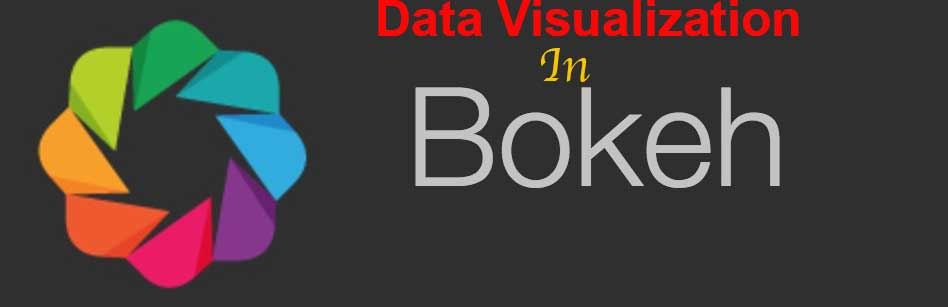•Almost one and half year back I create a data visualization series with Matplotlib which was appreciated by hundreds of developers. Today I am going to start another data visualization series to create interactive graphs and charts in Bokeh. What is Bokeh From the official website: Bokeh is an interactive visualization library that targets modern web browsers for presentation. Its goal is to provide elegant, concise construction of versatile graphics, and to extend this capability with high-performance interactivity over very large or streaming datasets. Bokeh can help anyone who would like to quickly and easily create interactive plots, dashboards, and data applications. In short, Bokeh allows Python developers to create…

• In this post I am going to discuss a Matplotlib feature which let you add multiple plots within a figure called subplots. Subplots are helpful when you want to show different data presentation in a single view, for instance Dashboards. There are multiple ways you can create subplots but I am here going to discuss the one which let you add graphs in grids by using subplot2grid method. subplot2grid takes two mandatory parameters, the first one is size and the next is location. A typical subplot2grid call will look like below: ax = plt.subplot2grid((2, 2), (0, 0)) The first parameter is the size that is a 2 x 2 grid, the 2nd is…

• In last post I discussed scatter, today I am going to discuss Pie charts. What are Pie Charts? An Emma chart (or a circle chart) is a circular statistical graphic which is divided into slices to illustrate numerical proportion. In a pie chart, the arc length of each slice (and consequently its central angle and area), is proportional to the quantity it represents. While it is named for its resemblance to a pie which has been sliced, there are variations on the way it can be presented. The earliest known pie chart is generally credited to William Playfair’s Statistical Breviary of 1801.   Pie charts are good to show proportional…

• In last post I talked about plotting histograms, in this post we are going to learn how to use scatter plots with data and why it could be useful. What is Scatter Plot? From Wikipedia: A scatter plot (also called a scatterplot, scatter graph, scatter chart, scattergram, or scatter diagram) is a type of plot or mathematical diagram using Cartesian coordinates to display values for typically two variables for a set of data. If the points are color-coded, one additional variable can be displayed. The data are displayed as a collection of points, each having the value of one variable determining the position on the horizontal axis and the value…

•   In the last post I talked about bar graphs and their implementation in Matplotlib. In this post I am going to discuss Histograms, a special kind of bar graphs. What is Histogram? From Wikipedia A histogram is an accurate graphical representation of the distribution of numerical data. It is an estimate of the probability distribution of a continuous variable (quantitative variable) and was first introduced by Karl Pearson. It is a kind of bar graph. To construct a histogram, the first step is to “bin” the range of values—that is, divide the entire range of values into a series of intervals—and then count how many values fall into each…

•   In last post I covered line graph. In this post I am going to show how to draw bar graph by using Matplotlib. Bar Graph What is bar graph? According to Wikipedia A bar chart or bar graph is a chart or graph that presents categorical data with rectangular bars with heights or lengths proportional to the values that they represent. The bars can be plotted vertically or horizontally.    So in short, bar graphs are good if you to want to present the data of different groups that are being compared with each other. For this post I have picked the data of movies genres released from 1995-2017. The source of data is here. import matplotlib.pyplot as plt import numpy…

•   I recently covered data gathering via scraping. Now I am going to cover how the data can be visualized. The best way to do is to plot graphs. Graphs makes it easier to see the relation between a data variable with other. There are various kinds of graphs available: Line, Bar, Chart, Histogram etc. Since we are dealing in Python, it provides a very good library for plotting cool graphs. It’s called Matplotlib. From the Official Site: Matplotlib is a Python 2D plotting library which produces publication quality figures in a variety of hardcopy formats and interactive environments across platforms. Installation The best way to install it by using…